Home > INT3 > Chapter 7 > Lesson 7.2.5 > Problem7-115

7-115.

Consider the graph at right. Homework Help ✎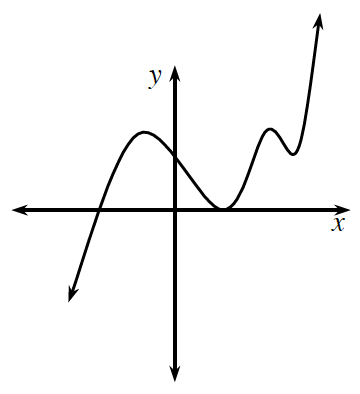1. Is the graph a function? Explain.

2. Sketch the inverse of this graph. Is the inverse a function? Justify your answer.

3. Must the inverse of a function be a function? Explain.

4. Describe a characteristic of functions that have inverse functions.

5. Could the inverse of a non-function be a function? Explain or give an example.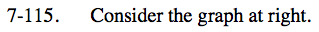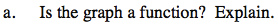What causes a graph to be a function or not a function?
Look up 'function' in the glossary if you aren't sure.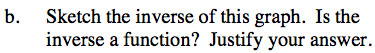Reflect the graph across y = x.
Are there any x-values that have more than one y-value?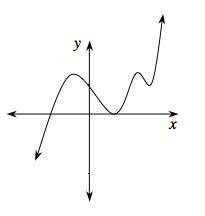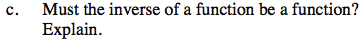Consider several different functions and their inverses.

See part (a).
Think about how this rule is translated to the inverse.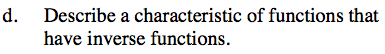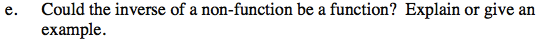Think about functions whose inverses are non-functions.
What about the inverse of that inverse?